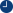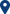# Force calculation between spherical particles fully or partially submerged in a liquid

07/01/2013:00-00:00Room EM204, ORT Braude College, Karmiel

MATHEMATICS COLLOQUIUM
=========================
Speaker: Dr. Anna Zigelman, Technion
Title: Force calculation between spherical particles fully or partially submerged in a liquid
Date & Time: Tuesday, January 7, 2020, at 13:00
Place: Room EM204, ORT Braude College, Karmiel

Abstract

I will discuss two cases for calculation of the potential between two spherical particles. Knowing the potential everywhere in space yields the force between the particles, which is needed e.g., for comparing theoretical models to the experimental results. In both cases we solved the Poisson – Boltzmann equation, which is an elliptic PDE, with Dirichlet boundary conditions on the surfaces of the particles.

In the first case, the particles were assumed to be fully submerged in the liquid, and since the potentials on their surfaces were assumed to be constant, resulting in an axi-symmetric problem, we used bi-polar coordinates system and solved the problem in 2D. Our results yielded nondimensional numbers which were used to analyze the experimental data obtained by our group member.

In the second case, the particles were assumed to be partially submerged in the liquid, so that exactly their half was assumed to be inside the liquid medium, namely below a flat liquid-gas interface. The liquid-gas interface was assumed to be charged and broke-up the axi-symmetry of the problem.

Thus, in this case we solved a linearized version of Poisson-Boltzmann equation in 3D. We used an iterative approach in order to determine the coefficients in a semi-analytic solution. Furthermore, we have approximated the force versus separation curves, which were obtained for different parametric values, by Páde function, thus allowing a simple use of our results by experimentalists.